Author: Oscar Cronquist Article last updated on May 14, 2022

The INDEX function returns a value from a cell range, you specify which value based on a row and column number.

Formula in cell C9:

=INDEX(B3:E7,2,3)

This formula returns a value from row 2 and column 3 based on cell range B3:E7, note these are relative positions.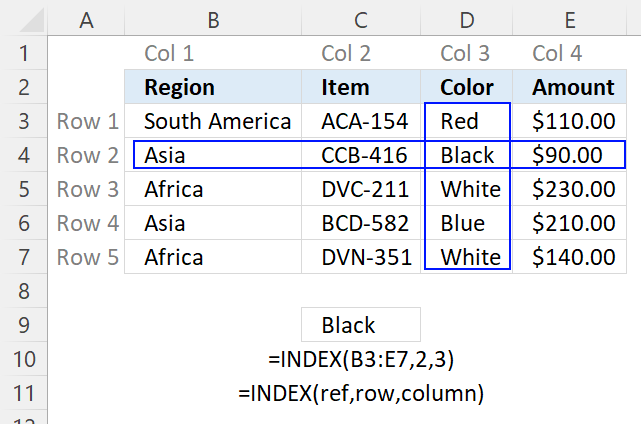The image above shows the relative row and column numbers, row 2 and column 3 are highlighted. The intersection of those two is the value the INDEX function returns.

## 1. Excel Function Syntax

INDEX(array, [row_num], [column_num], [area_num])

## 2. Arguments

 array or cell reference Required. The cell range you want to get a value from. You can also use an array. [row_num] Optional. The relative row number of a specific value you want to get. If omitted the INDEX function returns all values if you enter it as an array formula. Update! The 365  subscription version of Excel returns all values without needing to enter the formulas an array formula. [column_num] Optional. The relative column number of a specific value you want to get. If omitted the INDEX function returns all values if you enter it as an array formula. Update! The 365  subscription version of Excel returns all values without needing to enter the formulas an array formula. [area_num] Optional. A number representing the relative position of one of the ranges in the first argument.

## 3. How to use an array in INDEX function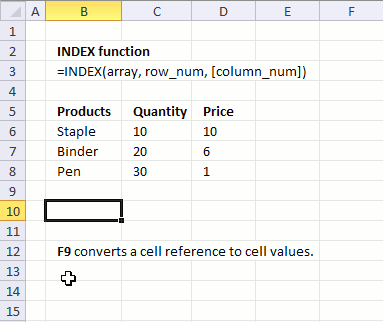The first argument in the INDEX function is array or a cell reference to a cell range. What is an array? An array is a range of values hardcoded into the formula.

To demonstrate in greater detail what an array is you can convert an array or a cell reference to a group of constants by selecting the cell reference and then press F9 to convert the cell reference to values, see the animated image above.

When you convert a cell range to constants Excel automatically creates double quotes around text values, however, note that numbers are not changed.

B6:D8 becomes {"Staple",10,10;"Binder",20,6;"Pen",30,1} and each value is separated by a delimiting character. Comma (,) is used to separate columns and semicolon (;) to separate rows.

The English language version of excel uses commas and semicolons, other language versions of excel may use other characters. You can change this in the Regional settings in Windows.

Here is an example of an array used in an INDEX function:

=INDEX({"Staple", 10, 10; "Binder", 20, 6; "Pen", 30, 1}, 3, 1)

The greatest disadvantage of using an array is that you need to edit the formula if you need to change one of the values in the array, contrary to a cell reference.

Here is an example of a cell reference being used in an INDEX function:

=INDEX(B6:D8, 3, 1)

You don't need to edit this formula if one of the values in cell range B6:D8 is changed, the formula is using the new value automatically.

Remember that relative cell references (B6:D8) changes when you copy the cell and paste to cells below. Absolute cell references (\$B\$6:\$D\$8) do not change when the cell is copied to cells below.

Read more about converting cell references or formulas: Replace a formula with its result

## 4. How to use the row_num argument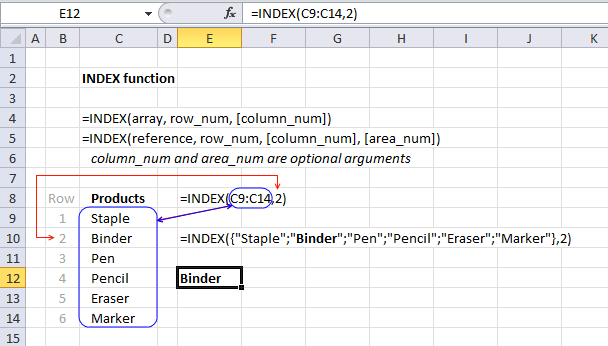The second argument in the INDEX function is the row_num. It allows you to choose the row in an array or cell range, from which to return a value.

If you use an array or cell range with values distributed in one column only there is no need to use the second optional argument which specifies the column, there is only one column to use. Here is an example of an array containing values in a single column, no comma as a delimiting value in this array which would have indicated that there would have been multiple columns.

=INDEX({"Staple"; "Binder"; "Pen"; "Pencil"; "Eraser"; "Marker"}, 2)

The following formula uses a cell reference instead of hardcoded values:

=INDEX(C9:C14, 2)

Cell range C9:C14 has values separated by a semicolon. The cell range is one-dimensional. In this example, the value from the second row will be returned, see image above.

## 5. Return an array of values - INDEX function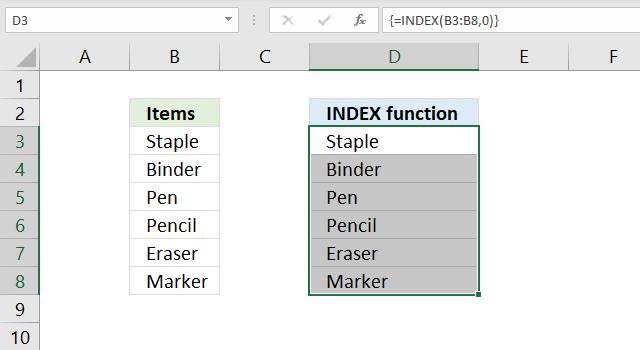It is also possible to return an array of values if you omit or use a zero as row_num argument:

=INDEX(C9:C14,)
=INDEX(C9:C14,0)

Both these formulas return an array of values. To be able to display all values you need to enter the formula as an array formula in a cell range that has the same number of cells as the cell range or values in the array.

1. Select cell range D3:D8.
2. Type the formula =INDEX(C9:C14,0)
3. Press and hold CTRL + SHIFT simultaneously.
4. Press Enter once.
5. Release all keys.

The formula in the formula bar changes to {=INDEX(C9:C14,0)}, do not add these curly brackets yourself, they appear automatically. See the image above.

Update 1/22/2020!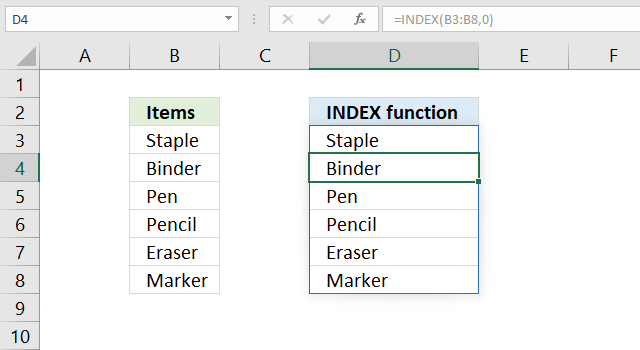Excel users owning Excel 365 subscription version now have the option to not enter the formula as an array formula but as a regular formula. They are called dynamic arrays and behaves differently than array formulas. Array formulas can still be used in order to be compatible with earlier Excel versions, however, Microsoft suggests that you should from now on use dynamic arrays instead of array formulas.

The formula is entered as a regular formula and extends automatically if the cells needed below are empty, this is called spilling by Microsoft. The remaining cells show a greyed out formula in the formula bar, only the first cell contains a formula in black.

The blue border around the cell range indicates that the cell range contains a spilled formula and disappears when you press with left mouse button on a cell outside the range.

## 6. How to use the [column_num] argument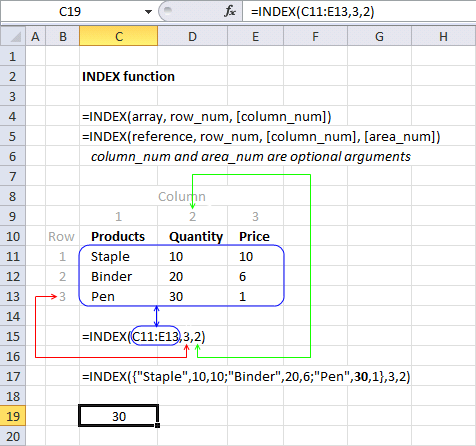The column_num argument allows you to choose a column from which to return a value. This argument is optional, for example, if you only have values in a single column.

The cell range C11:E13 is two-dimensional meaning there are multiple rows and columns. In this example, the value in the third row and the second column is returned.

=INDEX(C11:E13, 3, 2)

I have greyed out the row and column numbers in the image above, this makes it easier to see that value 30 is where row 3 and column 2 interesects.

The following formula has an array containing constants.

=INDEX({"Staple", 10, 10; "Binder", 20, 6; "Pen", 30, 1}, 3, 2)

{"Staple", 10, 10; "Binder", 20, 6; "Pen", 30, 1} has values separated by commas and semicolons meaning commas separate values between columns and semicolons separate values between rows.

## 7. How to use the [area_num] argument - INDEX function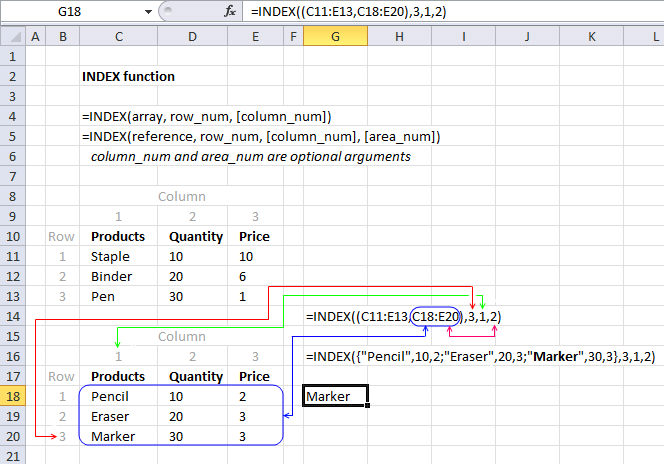The INDEX function lets you have multiple cell references in the first argument, the area_num argument allows you to pick a cell range in the reference argument.

INDEX(referencerow_num[column_num], [area_num])

The following formula has two references pointing to two different cell ranges.

=INDEX((C11:E13,C18:E20),3,1,2)

The area_num selects from which cell reference to return a value. In this example, area_num is two therefore the second cell reference is used. The item in the third row and the first column is returned.

## 8. How to return the entire row using the INDEX function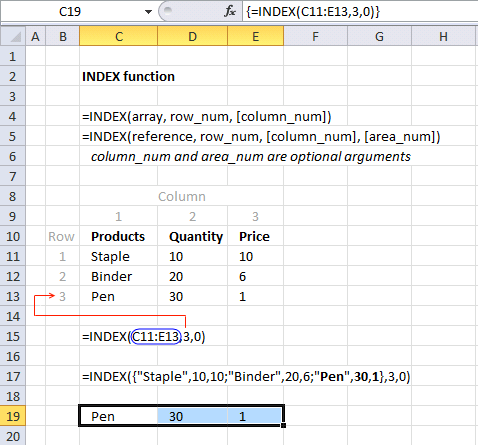The INDEX function is also capable of returning an array from a column, row, and both columns and rows. The following formula demonstrates how to extract all values from row three:

=INDEX(C11:E13,3,0)

The formula in cell C19:E19 is an array formula.

1. Select cell range C19:E19.
2. Type =INDEX(C11:E13,3,0) in formula bar.
3. Press and hold CTRL + SHIFT simultaeously.
4. Press Enter.
5. Release all keys.

### 8.1 How to return a column - INDEX function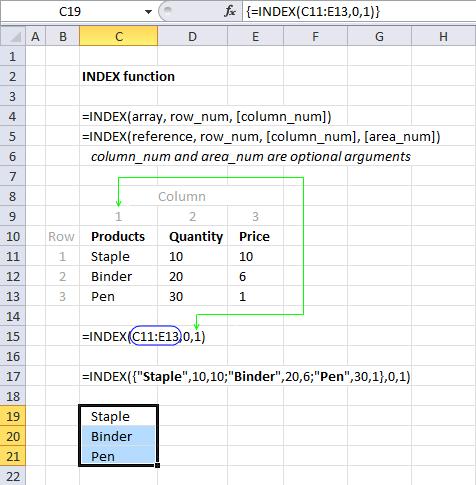The example above demonstrates an array formula that returns all values from column 1 from cell range C11:E13.

=INDEX(C11:E13, 0, 1)

### 8.2 How to return a two-dimensional cell range  - INDEX function

The example below returns all values from a two-dimensional cell range.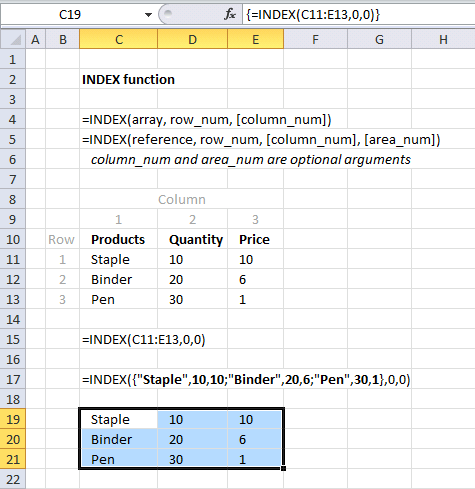The following array formula returns all values on all rows and columns from a cell range.

=INDEX(C11:E13,0,0)

## 9. How to build a dynamic cell reference using the INDEX function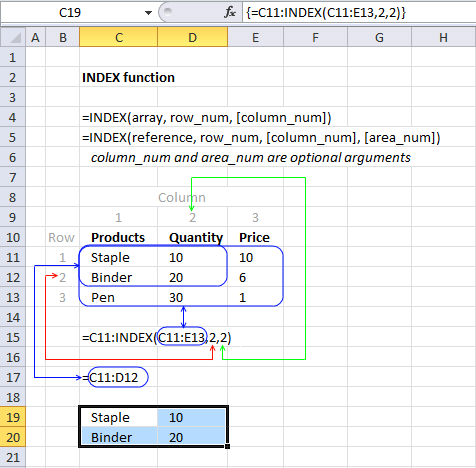The INDEX function can also be used to create a cell reference, for example, a dynamic range created by a formula in a named range.

Array formula in cell range C19:D20:

=C11:INDEX(C11:E13,2,2)

INDEX(C11:E13,2,2) returns cell reference D12

C11:D12 returns {"Staple",10;"Binder",20}

### Final note

There are some magic things you can do with the array argument. See this post: No more array formulas?